# Functions & Graphs: Age 14-16

This is part of our Secondary Curriculum collection of favourite rich tasks arranged by topic.### Back Fitter

##### Age 14 to 18Challenge Level

10 graphs of experimental data are given. Can you use a spreadsheet to find algebraic graphs which match them closely, and thus discover the formulae most likely to govern the underlying processes?### What's That Graph?

##### Age 14 to 18Challenge Level

Can you work out which processes are represented by the graphs?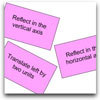### Surprising Transformations

##### Age 14 to 16Challenge Level

I took the graph y=4x+7 and performed four transformations. Can you find the order in which I could have carried out the transformations?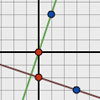### Perpendicular Lines

##### Age 14 to 16Challenge Level

Position the lines so that they are perpendicular to each other. What can you say about the equations of perpendicular lines?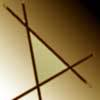### Negatively Triangular

##### Age 14 to 16Challenge Level

How many intersections do you expect from four straight lines ? Which three lines enclose a triangle with negative co-ordinates for every point ?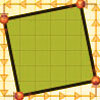### At Right Angles

##### Age 14 to 16Challenge Level

Can you decide whether two lines are perpendicular or not? Can you do this without drawing them?##### Age 14 to 16Challenge Level

In this problem we are faced with an apparently easy area problem, but it has gone horribly wrong! What happened?### Exploring Cubic Functions

##### Age 14 to 18Challenge Level

Quadratic graphs are very familiar, but what patterns can you explore with cubics?### Parabolic Patterns

##### Age 14 to 18Challenge Level

The illustration shows the graphs of fifteen functions. Two of them have equations y=x^2 and y=-(x-4)^2. Find the equations of all the other graphs.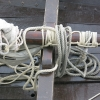### Tangled Trig Graphs

##### Age 16 to 18Challenge Level

Can you work out the equations of the trig graphs I used to make my pattern?### Functions and Graphs - Short Problems

##### Age 11 to 16

A collection of short problems on straight line graphs.### Curve Fitter

##### Age 14 to 18Challenge Level

This problem challenges you to find cubic equations which satisfy different conditions.You may also be interested in this collection of activities from the STEM Learning website, that complement the NRICH activities above.